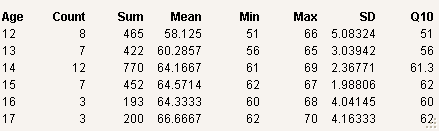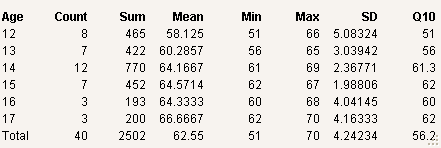For the latest version of JMP Help, visit JMP.com/help.

Scripting Guide > Data Tables > Advanced Data Table Scripting > Store Summary Statistics in Global Variables
Publication date: 07/30/2020

## Store Summary Statistics in Global Variables

The Summarize command collects summary statistics for a data table and stores them in global variables. The Summarize command is different from the Summary command, which also calculates summary statistics, but presents them in a new data table.

The first argument is an optional data table reference. Include it if more than one data table might be open.

`dt1 = Open( "\$SAMPLE_DATA/Big Class.jmp" );`
`dt2 = Open( "\$SAMPLE_DATA/Animals.jmp" );`
`Summarize( dt1,`
`	exg = By( :sex ),`
`	exm = Mean( :height )`
`);`
`Show( exg );`
`Show( exm );`

Named arguments include the following: Count, Sum, Mean, Min, Max, StdDev, First, Corr, and Quantile. These statistics can be calculated only for numeric columns. Each argument takes a data column argument.

Notes:

If a name=By(groupvar) statement is included, then a list of subgroup statistics is assigned to each name.

Count does not require a column argument, but it is often useful to specify a column to count the number of nonmissing values.

Quantile also takes a second argument for specifying which quantile, such as 0.1 for the 10th percentile.

When you summarize data that contain row states, the summary statistics are not calculated. An error appears in the log instead.

Note: Excluded rows are excluded from Summarize calculations. If all data are excluded, Summarize returns lists of missing values. If all data have been deleted (there are no rows), Summarize returns empty lists.

The following example uses the Big Class sample data table:

`dt = Open( "\$SAMPLE_DATA/Big Class.jmp" );`
`Summarize(`
`	a = By( :age ),`
`	c = Count,`
`	sumHt = Sum( :height ),`
`	meanHt = Mean( :height ),`
`	minHt = Min( :height ),`
`	maxHt = Max( :height ),`
`	sdHt = Std Dev( :height ),`
`	q10Ht = Quantile( :height, .10 )`
`);`
`Show( a, c, sumHt, meanHt, minHt, maxHt, sdHt, q10Ht );`

Because the script included a By group, the results are a list and six matrices:

a = {"12", "13", "14", "15", "16", "17"}

c = [8, 7, 12, 7, 3, 3]

sumHt = [465, 422, 770, 452, 193, 200]

meanHt = [58.125, 60.28571428571428, 64.16666666666667, 64.57142857142857, 64.33333333333333, 66.66666666666667]

minHt = [51, 56, 61, 62, 60, 62]

maxHt = [66, 65, 69, 67, 68, 70]

sdHt = [5.083235752381126, 3.039423504234876, 2.367712103711172, 1.988059594776032, 4.041451884327343, 4.163331998932229]

q10Ht = [51, 56, 61.3, 62, 60, 62]

You can format the results using Table Box.

`New Window( "Summary Results",`
`	Table Box(`
`		String Col Box( "Age", a ),`
`		Number Col Box( "Count", c ),`
`		Number Col Box( "Sum", sumHt ),`
`		Number Col Box( "Mean", meanHt ),`
`		Number Col Box( "Min", minHt ),`
`		Number Col Box( "Max", maxHt ),`
`		Number Col Box( "SD", sdHt ),`
`		Number Col Box( "Q10", q10Ht )`
`	)`
`);`

Figure 9.7 Results from SummarizeYou can add totals to the window, as follows:

`dt = Open( "\$SAMPLE_DATA/Big Class.jmp" );`
` `
`Summarize(`
`	a = By( :age ),`
`	c = Count,`
`	sumHt = Sum( :height ),`
`	meanHt = Mean( :height ),`
`	minHt = Min( :height ),`
`	maxHt = Max( :height ),`
`	sdHt = Std Dev( :height ),`
`	q10Ht = Quantile( :height, .10 )`
`);`
` `
`Summarize(`
`	tc = Count,`
`	tsumHt = Sum( :height ),`
`	tmeanHt = Mean( :height ),`
`	tminHt = Min( :height ),`
`	tmaxHt = Max( :height ),`
`	tsdHt = Std Dev( :height ),`
`	tq10Ht = Quantile( :height, .10 )`
`);`
` `
`Insert Into( a, "Total" );`
`c = c |/ tc;`
`sumHt = sumHt |/ tsumHt;`
`meanHt = meanHt |/ tmeanHt;`
`minHt = minHt |/ tminHt;`
`maxHt = maxHt |/ tmaxHt;`
`sdHt = sdHt |/ tsdHt;`
`q10Ht = q10Ht |/ tq10Ht;`
` `
`New Window( "Summary Results",`
`	Table Box(`
`		String Col Box( "Age", a ),`
`		Number Col Box( "Count", c ),`
`		Number Col Box( "Sum", sumHt ),`
`		Number Col Box( "Mean", meanHt ),`
`		Number Col Box( "Min", minHt ),`
`		Number Col Box( "Max", maxHt ),`
`		Number Col Box( "SD", sdHt ),`
`		Number Col Box( "Q10", q10Ht )`
`	)`
`);`

Figure 9.8 Summarize with TotalIf you do not specify a By group, the result in each name is a single value, as follows:

`dt = Open( "\$SAMPLE_DATA/Big Class.jmp" );`
`Summarize(`

// a = By( :age ),

`	c = Count,`
`	sumHt = Sum( :height ),`
`	meanHt = Mean( :height ),`
`	minHt = Min( :height ),`
`	maxHt = Max( :height ),`
`	sdHt = Std Dev( :height ),`
`	q10Ht = Quantile( :height, .10 )`
`);`
`Show( c, sumHt, meanHt, minHt, maxHt, sdHt, q10Ht );`

c = 40;

sumHt = 2502;

meanHt = 62.55;

minHt = 51;

maxHt = 70;

sdHt = 4.24233849397192;

q10Ht = 56.2;

Summarize supports multiple By groups. For example, in Big Class.jmp, proceed as follows:

`dt = Open( "\$SAMPLE_DATA/Big Class.jmp" );`
`Summarize( g = By( :age, :sex ), c = Count() );`
`Show( g, c );`

g = {"12", "12", "13", "13", "14", "14", "15", "15", "16", "16", "17", "17"}, {"F", "M", "F", "M", "F", "M", "F", "M", "F", "M", "F", "M"}}

c = [5,3,3,4,5,7,2,5,2,1,1,2]

If you specify a By group, the results are always matrices. Otherwise, the results are scalars.

Want more information? Have questions? Get answers in the JMP User Community (community.jmp.com).